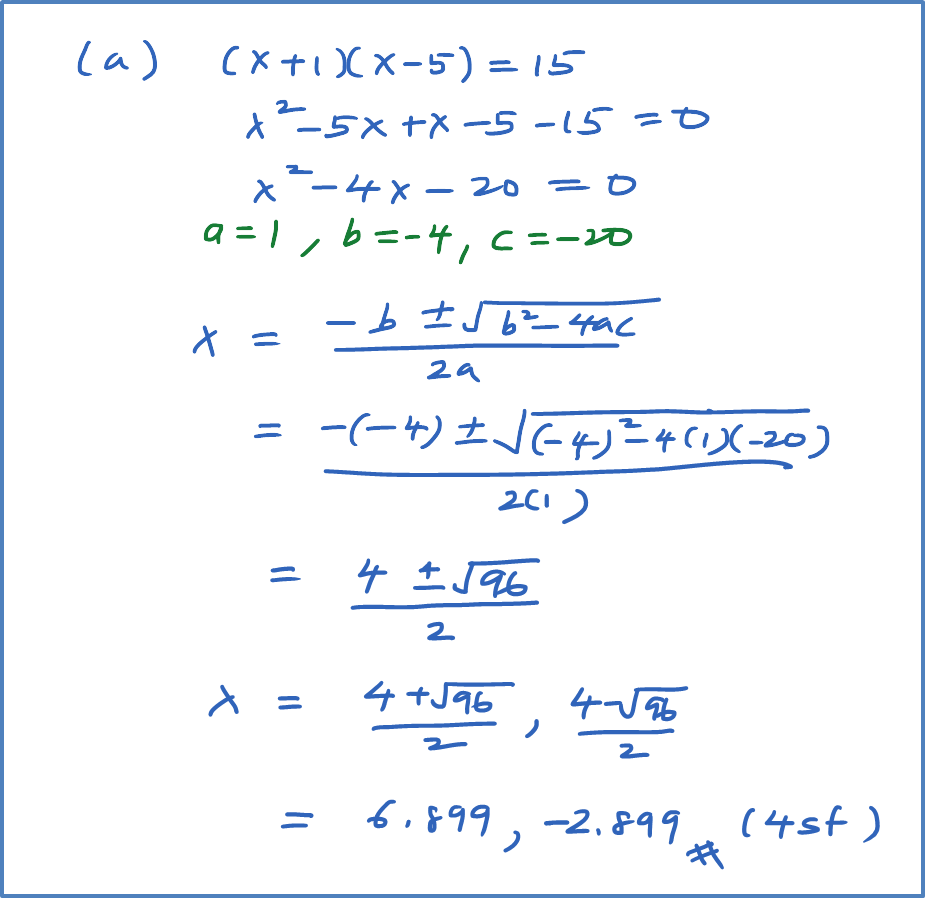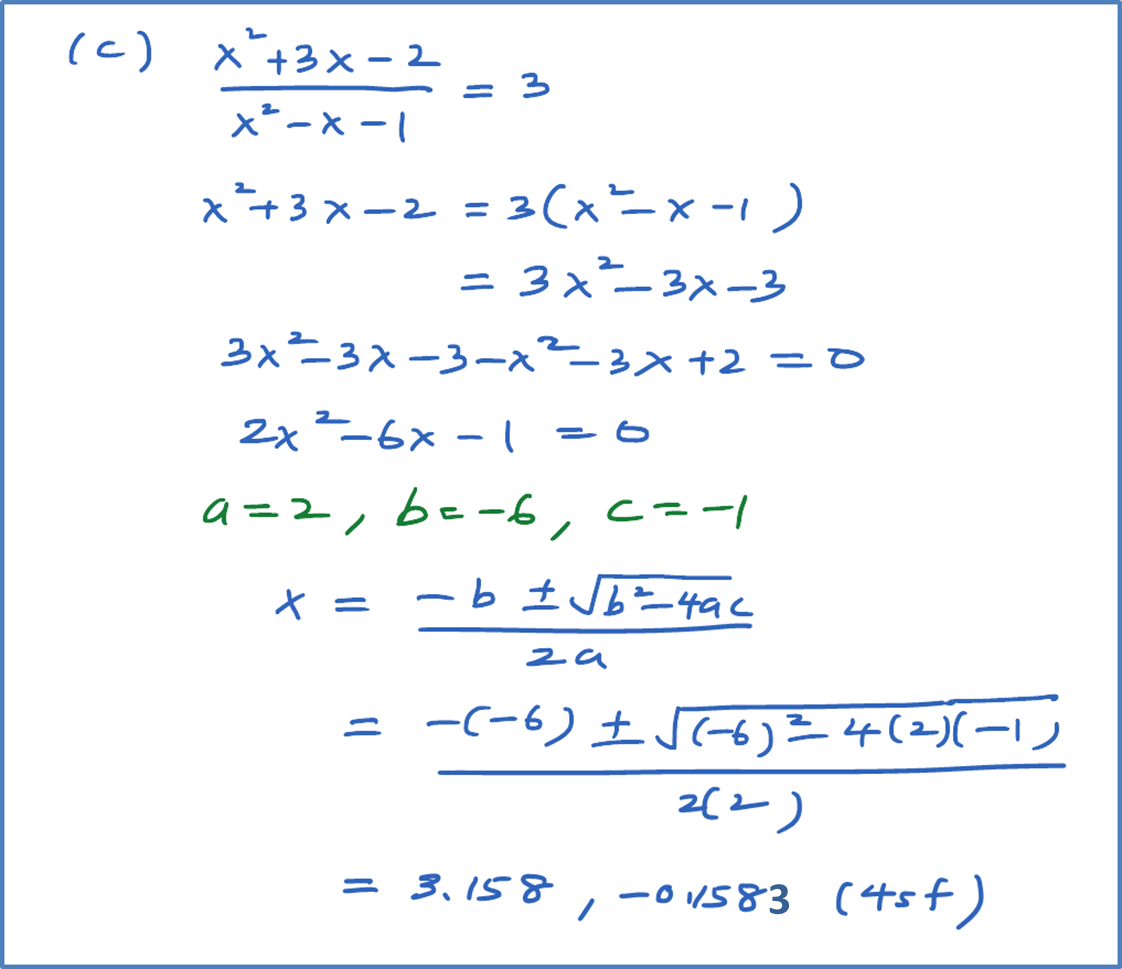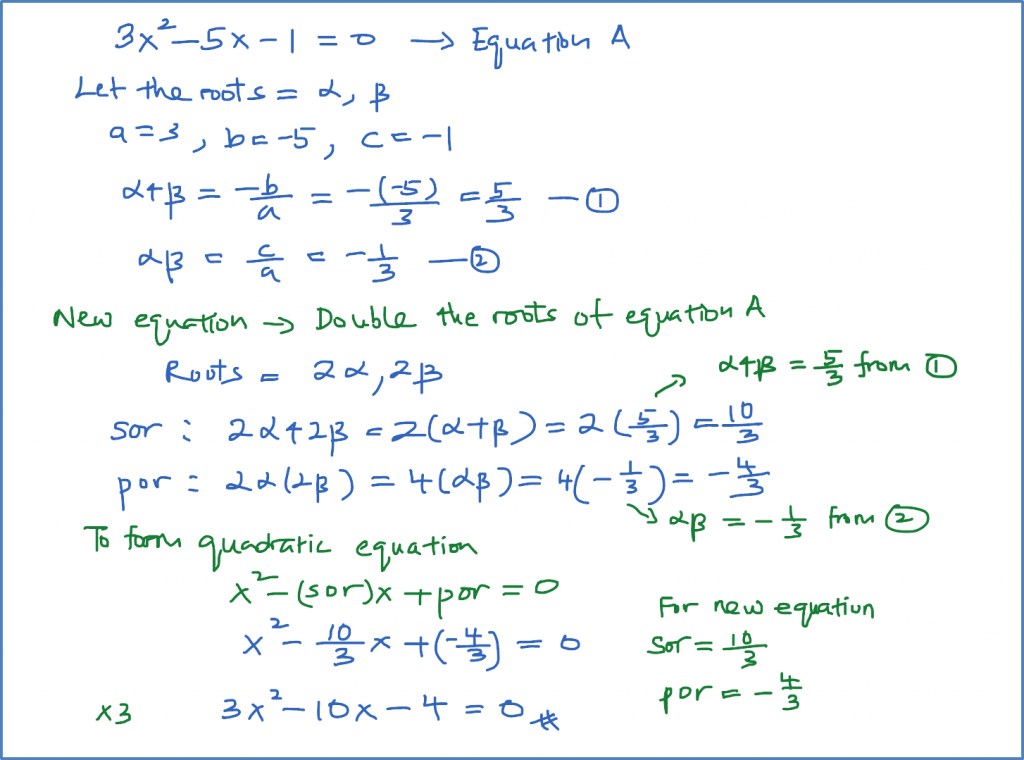2.9.1 Quadratic Equation, SPM Practice (Paper 1)

Question 1:
Solve the following quadratic equations by factorisation.

Solution:Question 2:
Solve the following quadratic equations by completing the square.

Solution:Question 3:
Solve the following quadratic equations by using quadratic formula. Give your answer in four significant figures.

Solution:Question 4:
If the roots of find the equations whose roots are

Solution:Question 5:
Write and simplify the equation whose roots are double the roots of $3{x}^{2}-5x-1=0$ , without solving the given equation.

Solution: